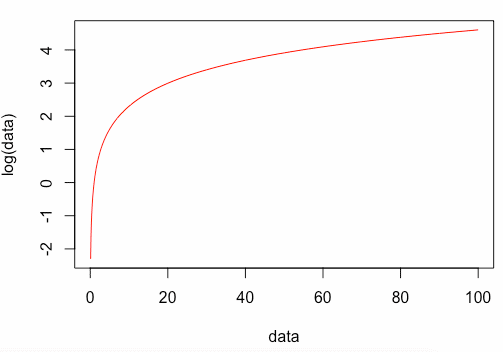The fundamental to calculate log in R is by using the log() method in the format of log(value, base) that returns the logarithm of the value in the base.

The log() is a built-in R function that calculates logarithms. It calculates natural logarithms by default. The log10() function calculates common (i.e., base 10) logarithms. The log2() function computes binary (i.e., base 2) logarithms.

The general form log(x, base) calculates logarithms with base.

The log1p(x) function calculates accurately also for |x| << 1.

The exp() function calculates the exponential function.

The expm1(x) function calculates accurately for |x| << 1.

### Syntax of log()

``log(x, base)``

### Parameters

1. x – It is numeric to which log has to be computed
2. base – It is the base of the log.

### Example

Let’s find the natural log of 11 using the log() function.

``````data <- log(11)
cat("The natural logarithm of 11 is: ")
cat(data)``````

#### Output

``The natural logarithm of 11 is: 2.397895``

## Plotting the log()

The most used plotting function in R programming is the plot() function. It is a generic function that has many methods called according to the type of object passed to the plot().

Open the rstudio and write the following code.

``````data <- seq(0, 100, by = 0.1)
plot(data, log(data), typ = "l", col = "red")``````

#### Output## Find the log of the vector sequence.

To find the log of vector sequence, pass the vector to the log() function. Before that, you have to create a vector sequence if it is not available to you. Let’s create a vector sequence for our example.

``````vc <- (rep(1:5))
cat("The vector sequence is: ")
cat(vc, "\n")``````

It will give us the following sequence.

``The vector sequence is: 1 2 3 4 5``

To find the log, pass the sequence to a log() function.

``````vc <- (rep(1:5))
cat("The vector sequence is: ")
cat(vc, "\n")
cat("Find the log() of vector sequence is: ")
cat(log(vc))
``````

#### Output

``````The vector sequence is: 1 2 3 4 5
Find the log() of vector sequence is: 0 0.6931472 1.098612 1.386294 1.609438
``````

## Specify the log base in R

You can specify the base of the log bypassing the second parameter to the log() function.

Let’s find the logarithmic value of the number or vector to the base 3.

``````data <- log(11, 3)
cat("The log() function in R with base 3: ")
cat(data)``````

#### Output

``The log() function in R with base 3: 2.182658``

Let’s find the log of vector sequence to the base 3.

``````vc <- (rep(1:5))
cat("The vector sequence is: ")
cat(vc, "\n")
cat("Find the log(vc, 3) of vector sequence is: ")
cat(log(vc, 3))
``````

#### Output

``````The vector sequence is: 1 2 3 4 5
Find the log(vc, 3) of vector sequence is: 0 0.6309298 1 1.26186 1.464974``````

## log2() function in R

To calculate the log value with the base 2, use the log2() function.

``````data <- log2(11)
cat("The log2() value of 11 is: ")
cat(data) ``````

#### Output

``The log2() value of 11 is: 3.459432``

Let’s find the log of vector sequence to the base 2.

``````vc <- (rep(1:5))
cat("The vector sequence is: ")
cat(vc, "\n")
cat("Find the log2() of vector sequence is: ")
cat(log2(vc))``````

#### Output

``````The vector sequence is: 1 2 3 4 5
Find the log2() of vector sequence is: 0 1 1.584963 2 2.321928``````

## log10() function in R

To calculate the log value with the base 10, use the log10() function.

``````data <- log10(11)
cat("The log10() value of 11 is: ")
cat(data)``````

#### Output

``The log10() value of 11 is: 1.041393``

Let’s find the log of vector sequence to the base 10.

``````vc <- (rep(1:5))
cat("The vector sequence is: ")
cat(vc, "\n")
cat("Find the log10() of vector sequence is: ")
cat(log10(vc))``````

#### Output

``````The vector sequence is: 1 2 3 4 5
Find the log10() of vector sequence is: 0 0.30103 0.4771213 0.60206 0.69897``````

## Find the log of the column in DataFrame

You can calculate the log of the data frame column in R using the log() function. Let’s create a data frame and calculate the log of one of the data frame’s columns.

``````gaming <- data.frame(console_name = c("Playstation", "XBOX S"),
console_price = c(399, 299),
game_name = c("Spiderman", "Forza Motors"))

print(gaming)``````

#### Output

``````    console_name        console_price      game_name
1   Playstation            399             Spiderman
2   XBOX S                 299             Forza Motors
``````

Let’s find the log values of the console_price column.

``````gaming <- data.frame(console_name = c("Playstation", "XBOX S"),
console_price = c(399, 299),
game_name = c("Spiderman", "Forza Motors"))

print(gaming)
gaming\$price_log <- log(gaming\$console_price)
print(gaming)``````

#### Output

``````     console_name   console_price     game_name
1    Playstation         399          Spiderman
2    XBOX S              299          Forza Motors
console_name    console_price    game_name     price_log
1    Playstation         399          Spiderman      5.988961
2    XBOX S              299          Forza Motors   5.700444``````

In this output, you can see that we have appended one more column called price_log, whose values are the log of a console_price column. So this is how you can use the log() function in R DataFrame.

That is it for the R log() function example.

Categories R